Author: Oscar Cronquist Article last updated on February 01, 2023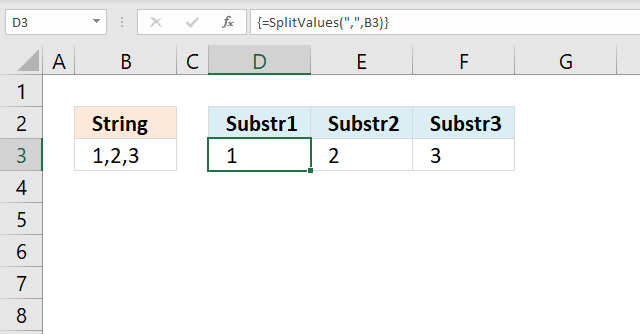The picture above shows a user-defined function (UDF) that splits the string in cell C3 using a delimiting character ",". It then returns an array of values to cell range D3:F3.

The SPLIT function is a function you can use in Visual Basic for Applications, abbreviation VBA.

It returns a one-dimensional array containing substrings from a divided string based on a delimiting character.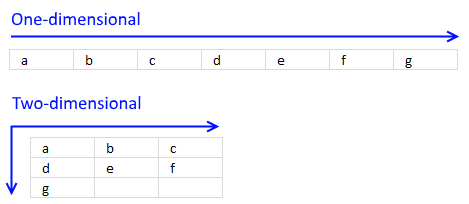The returning array is zero-based meaning the first substring starts with index 0 (zero), example below.

Array(0) = "a"
Array(1) = "b"
Array(2) = "c"
Array(3) = "d"

## 1. SPLIT Function Syntax - VBA

Split(expression ,[delimiter], [limit], [compare])

## 2. SPLIT Function Arguments - VBA

 expression Required. A string you want to split. [delimiter] Optional. The delimiting character, default value is " ", in other words, a space character. [limit] Optional. How many substrings to be returned, default value is all substrings. [compare] Optional. A numeric value determining how Excel compares when processing.

The following table shows the numeric values you can use in the compare parameter above.

 Constant Value Description vbUseCompareOption -1 Comparison using the setting of the Option Compare statement. vbBinaryCompare 0 Binary comparison vbTextCompare 1 Textual comparison vbDatabaseCompare 2 Microsoft Access only. Performs a comparison based on information in your database.

## 3. Example 1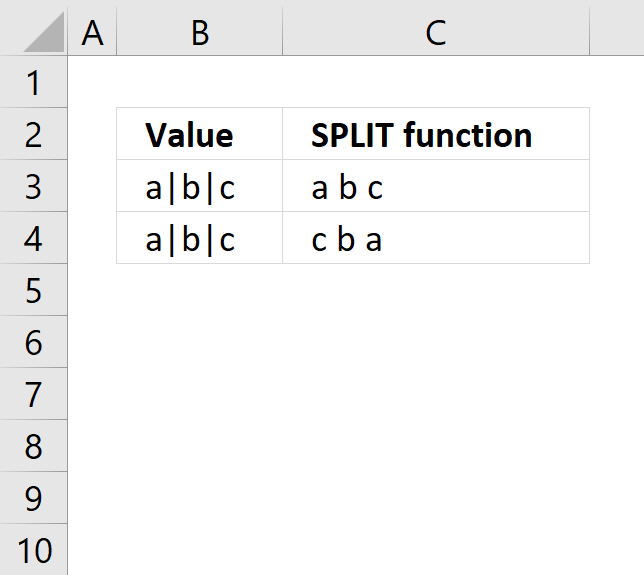The following UDF is demonstrated in the picture above in cell C3, it uses "|" to split the string in cell B3. The substrings are then concatenated with a space character between substrings. The returning value is shown in cell C3.

```'Name User Defined Function and arguments
Function SplitValues(rng As Range)

'Dimension variables and declare data types
Dim Arr() As String
Dim Str As Variant
Dim result As String

'Split value in variable Rng using delimiting character |
Arr = Split(rng, "|")

'Iterate through each substring
For Each Str In Arr

'Concatenate each substring and save to variable result
result = result & Str & " "
Next

'Return variable result to User Defined Function on worksheet
SplitValues = Trim(result)

End Function
```

## 4. Example 2The second UDF is shown in cell C4, it does the same thing as the first UDF above except it returns the last substrings first, in other words, substrings are concatenated backward.

```Function SplitValues1(rng As Range)
Dim Arr() As String
Dim Str As Variant
Dim result As String
Dim i As Integer

Arr = Split(rng, "|")

For i = UBound(Arr) To 0 Step -1
result = result & Arr(i) & " "
Next i

SplitValues1 = Trim(result)

End Function

```

## 5. Split cell value using a delimiting character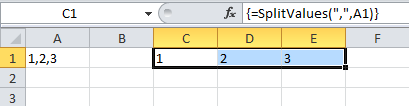The image above demonstrates a UDF in cell C1 that splits the cell value in cell A1 to substrings using a delimiting character.

The first argument is the delimiting character or characters, the second argument is a cell reference to a cell. The UDF is entered as an array formula if you use an Excel version that is older than Excel 365.

```'Name User Defined Function and arguments
Function SplitValues(a As String, b As String)

'Dimension variables and declare data types
Dim Text() As String

'Split variable b using variable a as the delimiting character, save to variable Text
Text = Split(b, a)

'Return output stored in variable Text to User Defined Function
SplitValues = Text
End Function
```

### 3.1 How to use it1. Select cell range C1:E1.
2. Type =SplitValues(",",A1) in the formula bar.
3. Press and hold CTRL + SHIFT simultaneously.
4. Press Enter once.
5. Release all keys.

If you did this right, the formula has now a leading { and an ending }, like this {=SplitValues(",",A1)}. They appear automatically, don't enter these characters yourself.

### 3.2 Explaining the user-defined function

#### Function name and arguments

A user defined function procedure always start with "Function" and then a name. This udf has two arguments, a and b. Both a and b are strings.

```Function SplitValues(a As String, b As String)
```

#### Declaring variables

```Dim Text() As String
```

Text() is a dynamic string array. Read more about Defining data types.

#### Split function

```Text = Split(b, a)
```

The Split function accepts a text string and returns a zero-based, one-dimensional array containing all sub strings. Split allows you also to specify a delimiting character, default is the space character. The substrings are stored as multiple strings in Text array.

#### The udf returns the substrings in Text

```SplitValues = Text
```

#### End a udf

```End function
```

A function procedure ends with the "End function" statement.

### 3.3 Where to put the code?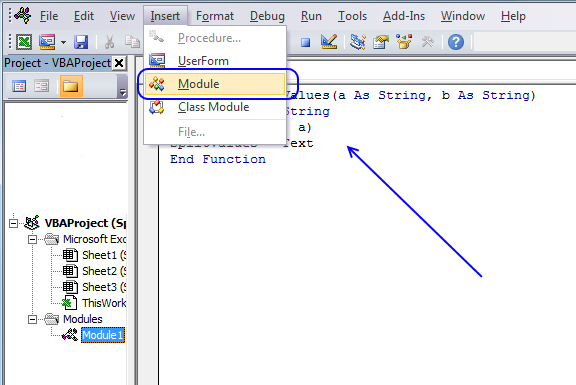To build a user-defined function, follow these steps:

1. Press Alt + F11 to open the visual basic editor
2. Press with left mouse button on "Insert" on the menu
3. Press with left mouse button on "Module"
4. Copy the code above and paste it to the code module.